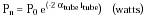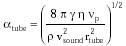Nanomedicine, Volume I: Basic Capabilities

Robert A. Freitas Jr., Nanomedicine, Volume I: Basic Capabilities, Landes Bioscience, Georgetown, TX, 1999

6.4.3.3 Hydraulic and Acoustic Tethers

Power is easily conveyed to medical nanodevices through tethers by simple hydraulic means. For example, a virtually leakproof thick-walled fullerene nanotube measuring 2rtube = 1 micron in diameter and ltube = 1 meter long could safely transport pressurized fluid of absolute viscosity h = 6.9 x 10-4 kg/m-sec (310 K water) at a fluid pressure ptube = 5 atm to drive a mechanical turbine or valved reciprocating piston system, establishing an energy density of 0.5 pJ/micron3 and delivering Pn = p rtube4 ptube2 / 8 h ltube ~ 10 pW to a Vn = 1 micron3 nanorobot power plant at a fluid flow velocity of vfluid = rtube2 ptube / 8 h ltube ~ 20 microns/sec and producing a power density of ~107 watts/m3, assuming Poiseuille flow.

Acoustic waves could be delivered by tether, but slightly larger tubes are required. Consider a water-filled pipe with dimensions as defined in the previous paragraph which is excited at one end by a vibrating piston operating at a frequency np << vsound/rtube (~1 GHz for 2rtube = 1 micron). For such small tubes, the inertia and kinetic reaction of the fluid may be neglected in favor of the frictional force (e.g., Poiseuille flow; Eqn. 9.25), since the compressions and rarefactions of the fluid are practically isothermal on account of the almost perfect heat conduction, and the power transmission is given by:{Eqn. 6.44}

with the attenuation coefficient given by Rayleigh's classical formulation:649,650{Eqn. 6.45}

where g is the ratio of specific heats (1.004 for water, 1.009 for seawater), vsound = 1500 m/sec and r = 993.4 kg/m3 for water at 310 K. Maximum energy transfer (like a rigid bar) occurs at integral multiples of half-wavelengths of the incident sonic waves, in other words, at np = n vsound / 2 ltube, where n = 1 is the fundamental frequency or first harmonic, n = 2 is the first overtone or second harmonic, and so forth. Minimum attenuation occurs at n = 1, the first harmonic.

A periodic source pulse of an intensity low enough to avoid cavitation in pure water (~104 watts/m2; Section 6.4.1) applied as input power P0 across a tube area of prtube2 produces a 10 pW output power for ltube < 300 microns at np = 2.5 MHz and rtube = 0.5 microns with P0 = 8000 pW and atube ~11,000 m-1. To get ltube = 1 meter length requires rtube = 15 microns and np = 750 Hz to deliver 10 pW at the output. (A three-phase "alternating current" acoustical power transmission system using 100 Hz waves traversing three water-filled pipes was patented by M. Constantinesco in 1920.)650

Diamond rods can also make nearly lossless acoustic power transmission lines (Section 7.2.5.3).

Last updated on 18 February 2003